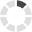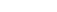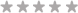Site InformationLoading... Please wait...
• My Account

FIN 370 Week 3 Assignment Question and Problem Set (Solutions)

\$15.00
Quantity:Product Description

Purpose of Assignment

Students should understand and be able to calculate the net present value and internal rate of return for corporate cash flows, determine project cash flows and a company's sales, variable costs, fixed costs, and its breakeven point.

Assignment Steps

Resources: Tutorial help on Excel® and Word® functions can be found on the Microsoft® Office website. There are also additional tutorials via the web that offer support for office products.

Complete the following Questions and Problems from each chapter as indicated.

Show all work and analysis.

Prepare in Microsoft® Excel® or Word.

Ch. 9: Questions 7 & 8 (Questions and Problems section)

7.   Calculating IRR  A firm evaluates all of its projects by applying the IRR rule. If the required return is 14 percent, should the firm accept the following project?

8.   Calculating NPV For the cash flows in the previous problem, suppose the firm uses the NPV decision rule. At a required return of 11 percent, should the firm accept this project? What if the required return is 24 percent?

Ch. 10: Questions 3 & 13 (Questions and Problems section)

3.   Calculating Projected Net Income  A proposed new investment has projected sales of \$635,000. Variable costs are 44 percent of sales, and fixed costs are \$193,000; depreciation is \$54,000. Prepare a pro forma income statement assuming a tax rate of 35 percent. What is the projected net income?

13.   Project Evaluation  Dog Up! Franks is looking at a new sausage system with an installed cost of \$540,000. This cost will be depreciated straight-line to zero over the project’s five-year life, at the end of which the sausage system can be scrapped for \$80,000. The sausage system will save the firm \$170,000 per year in pretax operating costs, and the system requires an initial investment in net working capital of \$29,000. If the tax rate is 34 percent and the discount rate is 10 percent, what is the NPV of this project?

Ch. 11: Questions 1 & 7 (Questions and Problems section)

1.  Calculating Costs and Break-Even  Night Shades, Inc. (NSI), manufactures biotech sunglasses. The variable materials cost is \$9.64 per unit, and the variable labor cost is \$8.63 per unit.

a. What is the variable cost per unit?

b. Suppose NSI incurs fixed costs of \$915,000 during a year in which total production is 215,000 units. What are the total costs for the year?

c. If the selling price is \$39.99 per unit, does NSI break even on a cash basis? If depreciation is \$465,000 per year, what is the accounting break-even point?

7.  Calculating Break-Even  In each of the following cases, calculate the accounting break-even and the cash break-even points. Ignore any tax effects in calculating the cash break-even.

Format your assignment consistent with APA guidelines if submitting in Microsoft® Word.

Product Reviews

This product hasn't received any reviews yet. Be the first to review this product!

Customers also viewed

• \$15.00• \$15.00• \$15.00• \$15.00• \$15.00Related Products

Click the button below to add the FIN 370 Week 3 Assignment Question and Problem Set (Solutions) to your wish list.